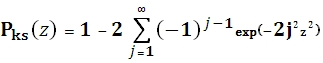# SQL Server Kolmogorov-Smirnov complementary cumulative distribution function

KSQ

Updated: 29 June 2012

Use KSQ to calculate the complementary Kolmogorov-Smirnov (KS) cumulative distribution function, Qks = 1 - Pks. The equation for the cumulative distribution function is:Syntax
SELECT [wctStatistics].[wct].[KSQ] (
<@z, float,>)
Arguments
@z
is the value at which to evaluate the function. @z is an expression of type float or of a type that can be implicitly converted to float
Return Types
Float
Remarks
·         @z must greater than or equal to 0
·         Qks(0) = 1
·         Qks(8) = 0
Examples
SELECT wct.KSQ(1) as KSQ
This produces the following result.
KSQ
----------------------
0.269999671677355

### SupportCopyright 2008-2023 Westclintech LLC         Privacy Policy        Terms of Service